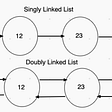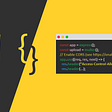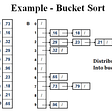# Why use the Sliding Window?

Nowadays, brute force quadratic time(O(N²)) solutions consisting of nested for loops just don’t cut it. The Sliding Window method is a great alternative to some brute force solutions. It typically of linear time(O(N)) complexity, and with space complexity of 0(1). If you are unfamiliar with time and space complexities check out Colton Kaiser’s articles on them here.

# What is the sliding window?

How we go about creating a sliding window utilizes pointers. We would get an array, assign the first pointer to one index signifying where the window starts, and the second pointer to another index signifying where the window ends. Between those two points gives us subsets of data we can work with, we can shift these points over to access other points within the array and create a new subset of data to check. just like how a window slides over, so does our subset of data. Hence the name Sliding Window! Here is an example question where we could make good use of the sliding window:

`Given an array of integers and a number, write a function which finds the maximum sum of a subarray with the length of the number passed to the function. Ifex:maxSubarraySum([]int{1, 1, 2, 4, 2, 3, 5, 1}, 4) //14maxSubarraySum([]int{100, 200, 300, 400}, 2) //700maxSubarraySum([]int{}, 2) //0`

Before we write any code, let's plan out the steps we want to take to come to a solution.

1. Write a check for the edge case if the array’s length is smaller than the subarray’s length. If it is then we return 0.
2. Assign a variable that will hold the highest sum(we’ll call it maxSum)
3. Assign a variable that will hold the sum of the current subarray we’re looking at. (we’ll call this tempSum)
4. Create a loop that will Loop through 1 time to get the sum of the first set of numbers
5. Set maxSum to equal the sum of our first loop and assign the tempSum to equal the current maxSum.
6. Loop through the length of the whole array starting from the index of the next number after the first set.
7. To get the sum of this set, instead of iterating we get the current tempSum move down the number of indexes equal to n, subtract that number, then add the number of the current loops index and assign that to be our new tempSum
8. Compare the new tempSum and the current maxSum, whichever of the two is larger is the new maxSum, and continue until the loop is finished.

Now that we have a plan in place let’s write it out!

# When should I use the Sliding Window?

Sliding Window won’t solve every problem but there are a lot of use cases for sliding window. Some good indicators that you might want to use the sliding window method are if it’s asking for the longest of shortest sequence, things involving a subarray like the example above, problems involving arrays and strings that you need to iterate through.

--

--

--

## More from Sean Dever

Love podcasts or audiobooks? Learn on the go with our new app.

## Hooks beyond React## Popup Share Modal UI Design using HTML CSS & JavaScript## JavaScript Math## A Solid RealWorld Demo Comparison of JavaScript Framework Performance## Building the Fitness Challenge App with Appwrite## Bucket Sort## Evaluation techniques for interactive systems## Apache SeaTunnel(Incubating) released version 2.1.1,## What is Virtualization in Cloud Computing?